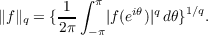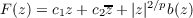#### Vol. 19, No. 2, 1966

 Download this articleFor screen For printingRecent Issues Vol. 325: 1  2 Vol. 324: 1  2 Vol. 323: 1  2 Vol. 322: 1  2 Vol. 321: 1  2 Vol. 320: 1  2 Vol. 319: 1  2 Vol. 318: 1  2Online Archive Volume: Issue:The Journal Subscriptions Editorial Board Officers Contacts Submission Guidelines Submission Form Policies for Authors ISSN: 1945-5844 (e-only) ISSN: 0030-8730 (print) Special Issues Author Index To Appear Other MSP Journals
Transformations of Fourier coefficients

### Daniel Rider

Vol. 19 (1966), No. 2, 347–355
##### Abstract

Let A and B be function spaces on the unit circle and let F be a complex function defined in the plane. F is said to map A into B provided F(an)ein𝜃 is the Fourier series of a function in B whenever anein𝜃 is the Fourier series of a function in A. For 1 q < , let Lq denote the usual space of functions on the unit circle normed by(1)

Let 2 q and p be given by p1 + q1 = 1.

It follows from the Hausdorff-Young theorem that if b(z) is bounded near the origin, then(2)

maps Lq into Lq.

In this paper it is shown that all functions mapping Lq into Lq have this form. In fact, all functions mapping the continuous functions into Lq have this form.

Theorem 1. Let 2 q . The following are equivalent.

(i) F maps Lq into Lq.

(ii) F maps the continuous functions into Lq.

(iii) F(z) = c1z + c2z + |z|2∕pb(z) where b(z) is bounded near the origin.

Primary: 42.10
##### Milestones